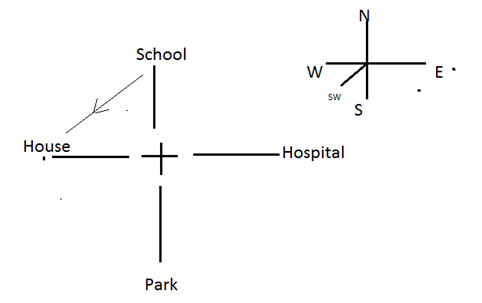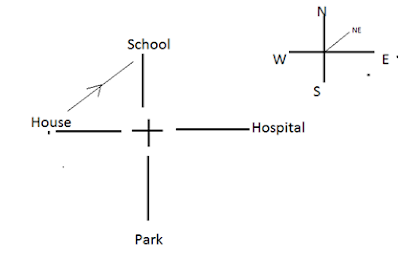New Student User - Use Code HELLO

# Direction Sense Test - Tricks and Shortcuts

Directions questions asked in the exam are based on two principles
1. Distance
2. Direction
You might be asked to calculate the distance or the direction. The foremost thing that you are supposed to do is draw basic direction map.For example, if you are asked to calculate the distance

### Ques 1. If I move 4 km towards east and then I travel 3 km towards the north. What is my distance from the starting point?

Remember your starting point is always the centre of the direction map.

Solution:
First of all, draw the map according to the directionsIt is a right angled triangle so by Pythagorean theorem
H2  =  P2 +B2
=  32 + 42
=  9 + 16
=  25
H = 5

So I am at a distance of 5 KM from the starting point.

Similarly, you can be asked to find the direction

### Ques 2. If I move 4 KM towards the east and then I travel 3 Km towards the north. In which direction I am from the starting point?

Solution:
Draw the map and try to superpose the direction map like thisNow it is quite clear from the map that I am in NE direction from the starting point.

This question can also be asked in a different way like :

In which direction I am from my final position ?,

Now scenario will change and the final position would act as the centre of the map.It is clear from the map I am in SW direction from my starting position.

There are a few thing that you need to keep in mind while solving these questions
1. Direction: N S E W
2. Left –Right
3. Straight / opposite
4. Sunrise and sunset

### Ques 3. A person starts from position A. He goes towards the east and then takes a right turn. Again the person takes a left turn and moves and then again he moves 45 degrees right. In which direction he is from the starting position?

Solution;
Consider yourself as the person and draw the map.It is clear that he is in the SE direction the starting point.

### Ques 4. A person P is standing at a point, another person Q is standing in the north of P. Q’s friend R is in right of Q and P’s friend S is standing left of P.In which direction R is standing with respect to S.

Solution:It is clear from the picture with respect to R, S is SW direction.

### Ques 5. In which direction is S with respect to R ?

It is clear that with respect to R, S is in the NE direction.

### Ques 6. I start from my house and go straight and come at a crossroad. The road which is coming from opposite direction ends at a hospital. When I walk from my house and turn right, there is a park on that road and opposite to park there is a school.What would be the direction of my house from the school?

Solution:
If it is straight, we can assume any direction.

Here the assumed direction is EAST.My house is in the SW from the school.

### Ques 7. What would be the direction of school from my house?

Solution:Clearly, school is NE direction from the school.

#### SUNRISE and SUNSET

Sun rises in the EAST then it shadow will be in the WEST.
Sun sets in the WEST then it shadow will be in the EAST.

### Ques 8. A man is facing towards the NORTH. At sunrise where will his shadow be? And if he is facing SOUTH. Then where will his shadow be?

Solution:If he is facing NORTH, his shadow will be in the WEST or in the left of the person.And If he is facing South, Then also his shadow will be in THE WEST or in the RIGHT of the person.